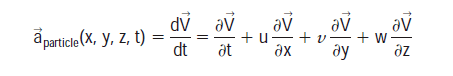# A simple differentiation and partial differentiation

• B
• mech-eng

#### mech-engHi, in the above why is the left-hand side simple differentiation, i.e V is only function of t but in the right it is function of t, x, y, and z. It is very strange that one side is different than the other. Would you like to explain it?

Thank you.

View attachment 99698

Hi, in the above why is the left-hand side simple differentiation, i.e V is only function of t but in the right it is function of t, x, y, and z. It is very strange that one side is different than the other. Would you like to explain it?

Thank you.
Mathematically it's clearly not right. It might be a useful exercise to figure out what would be a mathematically sound way to write out what is meant.

The key, I believe, is that the same symbol V is used for two different purposes.

$u=\frac{dx}{dt},\ v=\frac{dy}{dt},\ w=\frac{dz}{dt}$. Does this clarify?